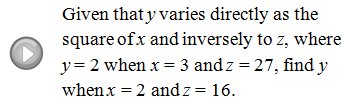## Pages

### Variation

Variation problems often set up as rational equations. Given two quantities x and y the following keywords indicate a particular relationship where k is called the variation constant.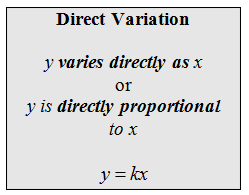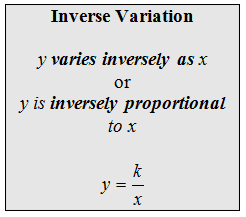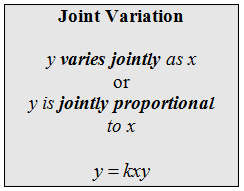Variation Problems
Example: Weight on Earth varies directly with the weight on the Moon. With his equipment, an astronaut weighs 360 pounds on earth but only 60 pounds on the Moon. If another astronaut had landed on the Moon that weighed 54 pounds with her equipment, how much would she weigh on Earth with equipment?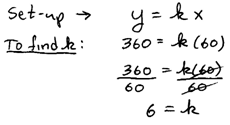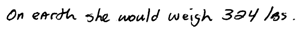Example: The weight of a body varies inversely as the square of its distance from the center of the Earth. If a person weighs 175 pounds on the surface of the earth (radius of the Earth = 4000 miles) how much will he weigh at 1,000 miles above the Earths surface?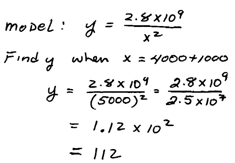Example: The distance d that a free-falling object has fallen is directly proportional to the square of the time that it has fallen.  An object falls 120 feet in 4 seconds. Find the constant of proportionality.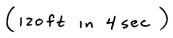Example: The area of an ellipse varies jointly as a and b, that is, half the major and minor axes.  If the area of an ellipse is 300 pi square units when a = 10 and b = 30 units then what is the constant of proportionality? Give a formula for the area of an ellipse.